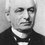# Three equations with square variables

Let

a^2+b^2+ab=9

b^2+c^2+bc=16

a^2+c^2+ac=25

What is the value of ab + bc +ac?Note by Sinuhé Ancelmo
6 years, 10 months ago

This discussion board is a place to discuss our Daily Challenges and the math and science related to those challenges. Explanations are more than just a solution — they should explain the steps and thinking strategies that you used to obtain the solution. Comments should further the discussion of math and science.

When posting on Brilliant:

• Use the emojis to react to an explanation, whether you're congratulating a job well done , or just really confused .
• Ask specific questions about the challenge or the steps in somebody's explanation. Well-posed questions can add a lot to the discussion, but posting "I don't understand!" doesn't help anyone.
• Try to contribute something new to the discussion, whether it is an extension, generalization or other idea related to the challenge.

MarkdownAppears as
*italics* or _italics_ italics
**bold** or __bold__ bold
- bulleted- list
• bulleted
• list
1. numbered2. list
1. numbered
2. list
Note: you must add a full line of space before and after lists for them to show up correctly
paragraph 1paragraph 2

paragraph 1

paragraph 2

[example link](https://brilliant.org)example link
> This is a quote
This is a quote
    # I indented these lines
# 4 spaces, and now they show
# up as a code block.

print "hello world"
# I indented these lines
# 4 spaces, and now they show
# up as a code block.

print "hello world"
MathAppears as
Remember to wrap math in $$ ... $$ or $ ... $ to ensure proper formatting.
2 \times 3 $2 \times 3$
2^{34} $2^{34}$
a_{i-1} $a_{i-1}$
\frac{2}{3} $\frac{2}{3}$
\sqrt{2} $\sqrt{2}$
\sum_{i=1}^3 $\sum_{i=1}^3$
\sin \theta $\sin \theta$
\boxed{123} $\boxed{123}$

Sort by:

Take a point $O$ from which three line segments $OA, OB, OC$, lying on the same plane, emanate such that their respective lengths are $a, b, c$ and the angle between any two of them is 120 degrees. Use Cosine rule and the mentioned equations to find $AB, BC, CA$ which will have values 3,4 and 5 units. Now this triangle $ABC$ is right angled( by the converse of Pythagoras' Theorem), so it's area will be 6 sq. units. Also, the area of the three triangles $AOB, BOC, COA$ can be found out by the sine rule (individually), and now equate it with the earlier found area. The answer comes out to be $8 \sqrt{3}$. [ the calculations are to be done by you].

- 6 years, 10 months ago

Well, your idea is interesting, but are you sure about the value of ab + bc +ac? shouldn' it be 8*sqrt(3)? Could you chek it again, plz??

- 6 years, 10 months ago

Edited.

- 6 years, 10 months ago

You can realize that - 8 * sqrt (3) is also a valid answer for ab + bc + ac, right?

- 6 years, 10 months ago

- 6 years, 10 months ago

Could you show any algebraic, only algebraic solution, friend?

- 6 years, 10 months ago

Is it possible to solve this algebraically?

- 6 years, 10 months ago

what will be the algebric solution Help me

- 5 years, 3 months ago

The solution could be algebraical or geometrical, Thanks in advance for help!

- 6 years, 10 months ago

The point "O" is known as "Fermat" or Torricelli Point of the triangle.

- 1 year, 12 months ago